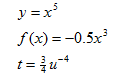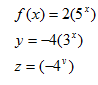Introduction

This unit will focus on two functions, power functions and exponential functions. They are put together because their equations look very similar, but their graphs are very different. A power function in general form is y = kxp, where k and p are constants. Some examples of power functions include:An exponential function in general form is y = abx, where a and b are constants. Since the equations are so similar, they are easy to confuse. The main difference between them is that the variable is in the exponent of the exponential function. This is the first instance where the variable has been in this position. Some examples of exponential functions include:Listen